JavaScript流程控制语句

if语句

if语句是基于条件成立才执行相应代码时使用的语句。

``````if(条件)
{ 条件成立时执行代码}``````

``````<script type="text/javascript">
var mycarrer = "HTML";
if (mycarrer == "HTML")
{
document.write("你面试成功，欢迎加入公司。");
}
</script>``````

if…else语句

if…else语句是在指定的条件成立时执行代码，在条件不成立时执行else后的代码。

``````if(条件)
{ 条件成立时执行的代码}
else
{条件不成立时执行的代码}``````

``````<script type="text/javascript">
var mycarrer = "HTML"; //mycarrer变量存储技能
if (mycarrer == "HTML")
{ document.write("你面试成功，欢迎加入公司。");  }
else  //否则，技能不是HTML
{ document.write("你面试不成功，不能加入公司。");}
</script>``````

if..else嵌套语句

``````if(条件1)
{ 条件1成立时执行的代码}
else  if(条件2)
{ 条件2成立时执行的代码}
...
else  if(条件n)
{ 条件n成立时执行的代码}
else
{ 条件1、2至n不成立时执行的代码}``````

``````var myscore = 86;
if(myscore < 60){
document.write("成绩不合格，加油了！")
}else if(myscore < 75){
document.write("成绩良好，不错啊")
}else if(myscore < 85){
document.write("成绩很好，很棒")
}else{
document.write("成绩优秀，超级棒棒")
}``````

Switch语句

``````switch(表达式)
{
case值1:
执行代码块 1
break;
case值2:
执行代码块 2
break;
...
case值n:
执行代码块 n
break;
default:
与 case值1 、 case值2...case值n 不同时执行的代码
}``````

Switch必须赋初始值，值与每个case值匹配。满足执行该 case 后的所有语句，并用break语句来阻止运行下一个case。如所有case值都不匹配，执行default后的语句。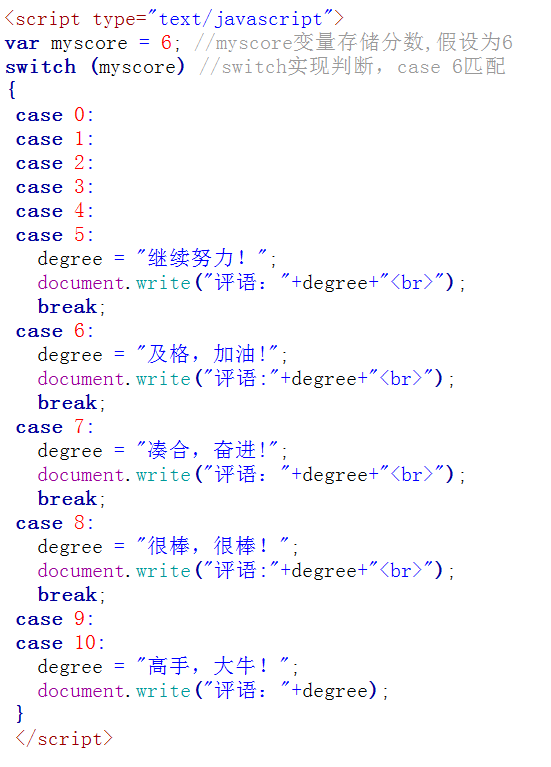``评语: 及格，加油!``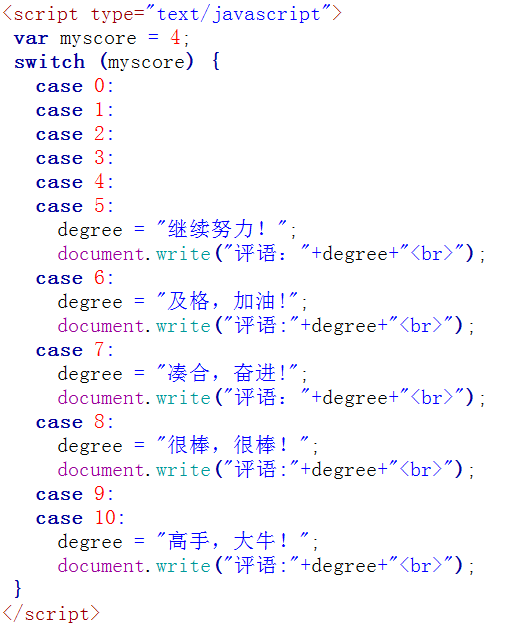``````评语: 继续努力!

for循环

for语句结构：

``````for(初始化变量;循环条件;循环迭代)
{
循环语句
}``````

``````<script type="text/javascript">
var num=1;
for (num=1;num<=6;num++)  //初始化值；循环条件；循环后条件值更新
{   document.write("取出第"+num+"个球<br />");
}
</script>``````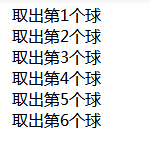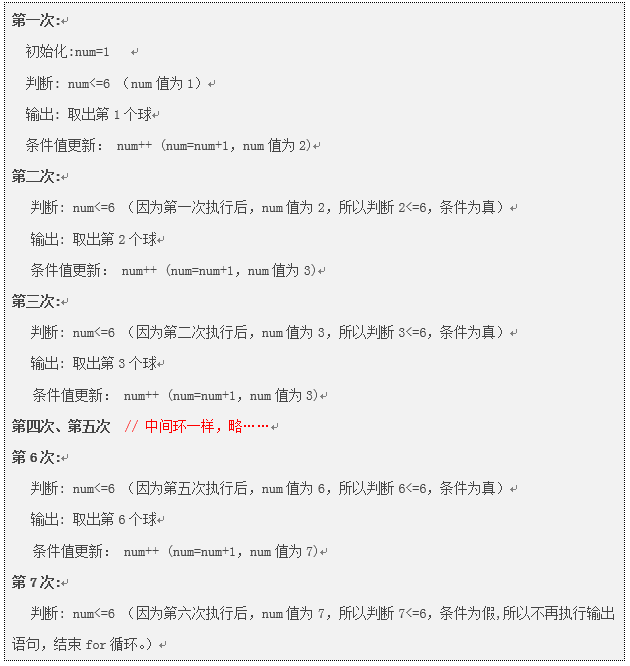while循环

while语句结构：

``````while(判断条件)
{
循环语句
}``````

``````var num=0;  //初始化值
while (num<=6)   //条件判断
{
document.write("取出第"+num+"个球<br />");
num=num+1;  //条件值更新
}``````

Do…while循环

do while结构的基本原理和while结构是基本相同的，但是它保证循环体至少被执行一次。因为它是先执行代码，后判断条件，如果条件为真，继续循环。

do…while语句结构：

``````do
{
循环语句
}
while(判断条件)``````

``````<script type="text/javascript">
num= 1;
do
{
document.write("数值为:" +  num+"<br />");
num++; //更新条件
}
while (num<=5)
</script>``````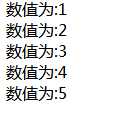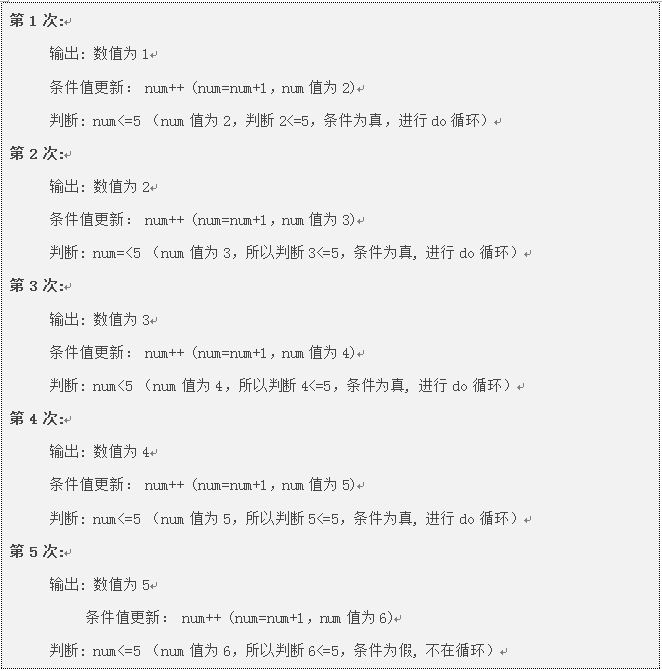退出循环break

``````for(初始条件;判断条件;循环后条件值更新)
{
if(特殊情况)
{break;}
循环代码
}``````

``````var num;
for(num = 1; num <= 10; num++){
if(num == 5){
break;//如果是5，退出循环
}
document.write("数值：" + num + "<br/>");
}``````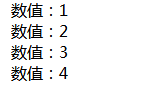继续循环continue

continue的作用是仅仅跳过本次循环，而整个循环体继续执行。

``````for(初始条件;判断条件;循环后条件值更新)
{
if(特殊情况)
{ continue; }
循环代码
}``````

``````var num;
for(num = 1; num <= 10; num++){
if(num == 5){
continue;//如果是5，退出循环
}
document.write("数值：" + num + "<br/>");
}``````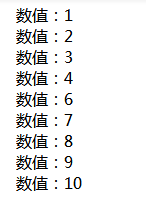编程练习

(‘小A’,’女’,21,’大一’),  (‘小B’,’男’,23,’大三’),

(‘小C’,’男’,24,’大四’),  (‘小D’,’女’,21,’大一’),

(‘小E’,’女’,22,’大四’),  (‘小F’,’男’,21,’大一’),

(‘小G’,’女’,22,’大二’),  (‘小H’,’女’,20,’大三’),

(‘小I’,’女’,20,’大一’),  (‘小J’,’男’,20,’大三’)

``````var infos = [
['小A','女',21,'大一'],
['小B','男',23,'大三'],
['小C','男',24,'大四'],
['小C','男',24,'大四'],
['小D','女',21,'大一'],
['小E','女',22,'大四'],
['小F','男',21,'大一'],
['小G','女',22,'大二'],
['小H','女',20,'大三'],
['小I','女',20,'大一'],
['小J','男',20,'大三']
];
//进行筛选
for(var i = 0; i < infos.length; i++) {
if(infos[i] === "大一" && infos[i] === "女") {
document.write(infos[i] + "<br />");
}
}``````

`````` //第一步把之前的数据写成一个数组的形式,定义变量为 infos
var infos = [
['小A','女',21,'大一'],
['小B','男',23,'大三'],
['小C','男',24,'大四'],
['小C','男',24,'大四'],
['小D','女',21,'大一'],
['小E','女',22,'大四'],
['小F','男',21,'大一'],
['小G','女',22,'大二'],
['小H','女',20,'大三'],
['小I','女',20,'大一'],
['小J','男',20,'大三']
];

var arr=new Array();
var n=0;
for(var i=0;i<infos.length;i++){
if(infos[i]=='大一'){
document.write(infos[i]+"<br />");  //第一次筛选，找出都是大一的信息
arr[n]=infos[i];
n++;
}
}
document.write("<br>"+"第二次筛选："+"<br>");
for(var n=0;n<arr.length;n++){
if(arr[n]=='女'){
document.write(arr[n]+"<br/>");//第二次筛选，找出都是女生的信息
}
}   ``````

觉得文章有用就打赏一下文章作者

支付宝扫一扫打赏微信扫一扫打赏在线客服

•扫描二维码，微信联系